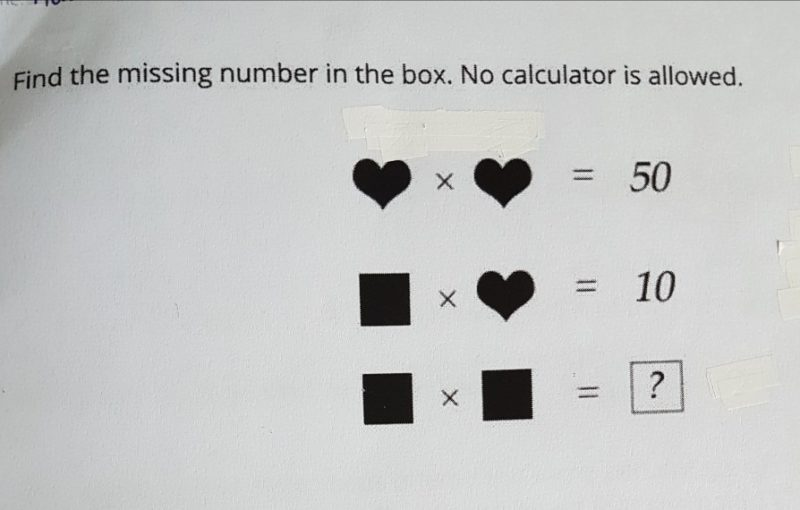# Questionhi, can help with this qn? tq!

Heart  x Heart = 50    -> (1)

Square  x Heart = 10   -> (2)

Divide (2) by (1),

Square / Heart = 1/5   -> (3)

Multiply (3) by itself

( Square / Heart )  x ( Square / Heart ) = 1/5 x 1/5 = 1/25

(Square x Square) / (Heart x Heart) = 1/25

Square x Square = 1/25 x (Heart x Heart) = 1/25 x 50 = 2